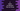# C program to delete an element from an Array## C program to delete an element from an Array:

In this post, we will learn how to delete an element from an Array in C. There is no method available to delete an element directly from an Array in C. We have to shift all elements one position left to do that.

Let’s learn how to do that.

### Algorithm to delete an element from an Array in C:

Our program will get the elements of the array as input from the user. It will also take the index of the element as another input.

We will follow the following algorithm to delete an element from an Array:

• Get the total number of array elements as input from the user.
• Create an array of that size.
• Ask the user to enter the elements of that array one by one. Read and store the elements in that array.
• Ask the user to enter the index of the element that needs to be deleted from the array.
• If the index is not a valid index, print a message to the user.
• If the index is valid, copy all elements from the right of it to one position left. Do it to the end of the array.
• Decrement the size of the array by 1

### C program to delete an element:

Below is the complete C program:

``````#include <stdio.h>

int main()
{
// 1
int arrSize, i, deleteIndex;

// 2
printf("Enter the size of the array: ");
scanf("%d", &arrSize);

// 3
int arr[arrSize];

for (i = 0; i < arrSize; i++)
{
printf("Enter the element for index %d: ", i);
scanf("%d", &arr[i]);
}

// 4
printf("Array: ");
for (i = 0; i < arrSize; i++)
{
printf("%d ", arr[i]);
}
printf("\n");

// 5
printf("Enter the element index to delete from the array: ");
scanf("%d", &deleteIndex);

if (deleteIndex < 0 || deleteIndex >= arrSize)
{
// 6
}
else
{
// 7
for (i = deleteIndex + 1; i < arrSize; i++)
{
arr[i - 1] = arr[i];
}

arrSize = arrSize - 1;

printf("Array: ");
for (i = 0; i < arrSize; i++)
{
printf("%d ", arr[i]);
}
}

printf("\n");

return 0;
}``````

#### Explanation:

The commented numbers in the above program denote the step numbers below:

1. Initialize three integer variables: arrSize to hold the size or length of the array, deleteIndex to hold the index of the element to delete from the array and i to use in the loops.
2. Ask the user to enter the size of the array. Read and store this value in the arrSize variable.
3. Create an array of size arrSize. By using a for loop, read the array elements from the user.
4. Print the array elements by using a for loop.
5. Ask the user to enter the index of the element to delete from the array. Read and store it in the deleteIndex variable.
6. If the value of deleteIndex not valid, print a message to the user.
7. If the value of deleteIndex is valid, run one for loop to copy all the elements to its left starting from deleteIndex + 1 to the last element.
8. Once the copy is done, subtract 1 from arrSize to update the new length. Print the new array elements after that.

#### Sample output:

It will print output as like below:

``````Enter the size of the array: 5
Enter the element for index 0: 1
Enter the element for index 1: 2
Enter the element for index 2: 3
Enter the element for index 3: 4
Enter the element for index 4: 5
Array: 1 2 3 4 5
Enter the element index to delete from the array: 4
Array: 1 2 3 4 ``````

### Method 2: By using separate methods:

We can also use separate methods. This increases the readability of the program and also we can remove all repeated code.

``````#include <stdio.h>

void printArray(int arr[], int size)
{
printf("Array: ");
for (int i = 0; i < size; i++)
{
printf("%d ", arr[i]);
}
printf("\n");
}

{
for (int i = 0; i < size; i++)
{
printf("Enter the element for index %d: ", i);
scanf("%d", &arr[i]);
}
}

void deleteItems(int arr[], int size, int deleteIndex)
{
if (deleteIndex < 0 || deleteIndex >= size)
{
}
else
{
for (int i = deleteIndex + 1; i < size; i++)
{
arr[i - 1] = arr[i];
}

size = size - 1;

printArray(arr, size);
}
}

int main()
{
int arrSize, i, deleteIndex;

printf("Enter the size of the array: ");
scanf("%d", &arrSize);

int arr[arrSize];

printArray(arr, arrSize);

printf("Enter the element index to delete from the array: ");
scanf("%d", &deleteIndex);

deleteItems(arr, arrSize, deleteIndex);

return 0;
}``````

Here,

• printArray is used to print an array. It removes all duplicate code from the first program.